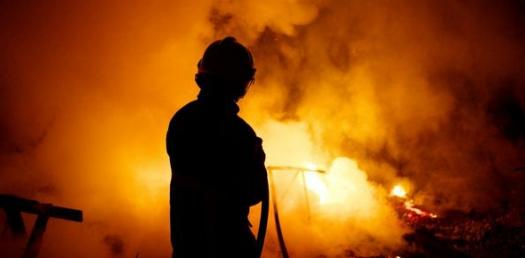# Fire Science And Fire Behavior

10 Questions | Total Attempts: 756SettingsThis quiz is 10 questions testing your knowledge of fire science and behavior

• 1.
What describes a process or reaction that releases energy usually in the form of heat, but also in the form of light (e.g. a spark, flame, or explosion), electricity (e.g. a battery), or sound?
• A.

Endothermic

• B.

Flash point

• C.

Exothermic

• D.

Ectothermic

• E.

Fire triangle

• 2.
An addition to the fire triangle. It adds the requirement for the presence of the chemical reaction which is the process of fire.
• A.

fire tetrahedron

• B.

exothermic reaction

• C.

Combustion

• D.

Exothermic

• E.

Endothermic

• 3.
The sequence of exothermic chemical reactions between a fuel and an oxidant accompanied by the production of heat and conversion of chemical species.
• A.

Oxidizer

• B.

Chain reaction

• C.

Combustion

• D.

• E.

Conduction

• 4.
Describes a process or reaction that absorbs energy in the form of heat
• A.

Exothermic

• B.

Endothermic

• C.

Ecothermic

• D.

enthalpy

• E.

convection

• 5.
The process of energy transfer from one body or system due to thermal contact, which in turn is defined as an energy transfer to a body in any other way than due to work performed on the body
• A.

Temperature

• B.

Convection

• C.

Conduction

• D.

• E.

Heat

• 6.
What is the percent of oxygen that sustaines life here on earth
• A.

35%

• B.

21%

• C.

18%

• D.

14%

• E.

7%

• 7.
Heat that is tranfered by the movement of molecules within fluids (i.e. liquids, gases and rheids).
• A.

• B.

Convection

• C.

Conduction

• D.

Combustion

• E.

Oxidation

• 8.
heat emitted from the surface of an object which is due to the object's temperature is what type of heat?
• A.

Thermal

• B.

• C.

Convection

• D.

Conduction

• E.

Combustion

• 9.
The chemical decomposition of condensed substances by heating, that occurs spontaneously at high enough temperatures
• A.

Combustion

• B.

Conduction

• C.

Gas pressure

• D.

Pyrolysis

• E.

Oxidation

• 10.
Is a traditional unit of energy. It is approximately the amount of energy needed to heat one pound of water one degree Fahrenheit.
• A.

BTU

• B.

International System of Units (SI)

• C.

Mass

• D.

Ectoplasma

• E.

Weight

Related TopicsBack to top